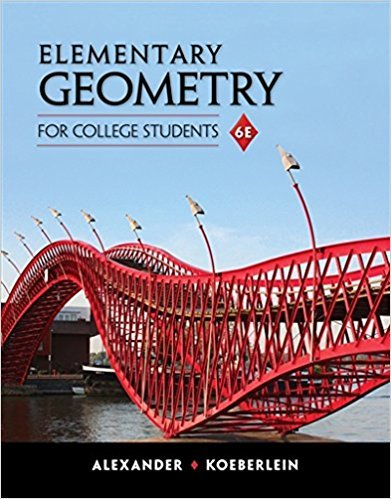×
×

# Solutions for Chapter 2: Parallel Lines## Full solutions for Elementary Geometry for College Students | 6th Edition

ISBN: 9781285195698Solutions for Chapter 2: Parallel Lines

Solutions for Chapter 2
4 5 0 310 Reviews
27
3
##### ISBN: 9781285195698

This textbook survival guide was created for the textbook: Elementary Geometry for College Students, edition: 6. Since 50 problems in chapter 2: Parallel Lines have been answered, more than 4221 students have viewed full step-by-step solutions from this chapter. This expansive textbook survival guide covers the following chapters and their solutions. Chapter 2: Parallel Lines includes 50 full step-by-step solutions. Elementary Geometry for College Students was written by and is associated to the ISBN: 9781285195698.

Key Math Terms and definitions covered in this textbook
• Adjacency matrix of a graph.

Square matrix with aij = 1 when there is an edge from node i to node j; otherwise aij = O. A = AT when edges go both ways (undirected). Adjacency matrix of a graph. Square matrix with aij = 1 when there is an edge from node i to node j; otherwise aij = O. A = AT when edges go both ways (undirected).

• Cramer's Rule for Ax = b.

B j has b replacing column j of A; x j = det B j I det A

• Cyclic shift

S. Permutation with S21 = 1, S32 = 1, ... , finally SIn = 1. Its eigenvalues are the nth roots e2lrik/n of 1; eigenvectors are columns of the Fourier matrix F.

• Distributive Law

A(B + C) = AB + AC. Add then multiply, or mUltiply then add.

• Dot product = Inner product x T y = XI Y 1 + ... + Xn Yn.

Complex dot product is x T Y . Perpendicular vectors have x T y = O. (AB)ij = (row i of A)T(column j of B).

• Free variable Xi.

Column i has no pivot in elimination. We can give the n - r free variables any values, then Ax = b determines the r pivot variables (if solvable!).

• Gram-Schmidt orthogonalization A = QR.

Independent columns in A, orthonormal columns in Q. Each column q j of Q is a combination of the first j columns of A (and conversely, so R is upper triangular). Convention: diag(R) > o.

• Identity matrix I (or In).

Diagonal entries = 1, off-diagonal entries = 0.

• Indefinite matrix.

A symmetric matrix with eigenvalues of both signs (+ and - ).

• Kronecker product (tensor product) A ® B.

Blocks aij B, eigenvalues Ap(A)Aq(B).

• Left nullspace N (AT).

Nullspace of AT = "left nullspace" of A because y T A = OT.

• Minimal polynomial of A.

The lowest degree polynomial with meA) = zero matrix. This is peA) = det(A - AI) if no eigenvalues are repeated; always meA) divides peA).

• Orthogonal subspaces.

Every v in V is orthogonal to every w in W.

• Orthonormal vectors q 1 , ... , q n·

Dot products are q T q j = 0 if i =1= j and q T q i = 1. The matrix Q with these orthonormal columns has Q T Q = I. If m = n then Q T = Q -1 and q 1 ' ... , q n is an orthonormal basis for Rn : every v = L (v T q j )q j •

• Schur complement S, D - C A -} B.

Appears in block elimination on [~ g ].

• Stiffness matrix

If x gives the movements of the nodes, K x gives the internal forces. K = ATe A where C has spring constants from Hooke's Law and Ax = stretching.

• Symmetric factorizations A = LDLT and A = QAQT.

Signs in A = signs in D.

• Toeplitz matrix.

Constant down each diagonal = time-invariant (shift-invariant) filter.

• Tridiagonal matrix T: tij = 0 if Ii - j I > 1.

T- 1 has rank 1 above and below diagonal.

• Vector v in Rn.

Sequence of n real numbers v = (VI, ... , Vn) = point in Rn.

×